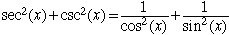I am stuck on a problem and wondering if you can help?? It is: Prove the following: sec2(X)+csc2(X) = sec2(X)csc2(X) Thank you! Debby Hi Debby, I find it easier to think about trigonometry problems if they are in terms of sines and cosines. sec(x) = 1/cos(x) and csc(x) = 1/sin(x), henceFind a common denominator for the two fractions and simplify. Does this help? Harley Go to Math Central